# gauss_kernel

Below is a demonstration of the features of the gauss_kernel function

## Contents

```clear; close all; clc;
```

## Syntax

hg=gauss_kernel(k,nd,f,m);

## Description

This function creates a Gaussian filtering kernel. The inputs are: k = the kernel size nd = the number of dimensions e.g. 1 for 1D, 2 for 2D, 3 for 3D etc. f = the Gaussian bell curve width measure, either the sigma (standard deviation) or the width methodOption = 'sigma' or 'width. If 'sigma' is choosen then f is interpretet as a Gaussian sigma. If 'width is used instead then f is interpretet as the point "where the bell curve is" at the edges of the kernel e.g. f=2 results in twice the standard deviation.

## Examples

Plot settings

```lineWidth=3;
```

## Example 1: 1D 'sigma' method

```k=25;
nd=1;
f=2;
methodOption='sigma';
hg=gauss_kernel(k,nd,f,methodOption);
```
```cFigure;
plot(hg,'r-','LineWidth',lineWidth);
axis tight; box on; grid on;
drawnow;
```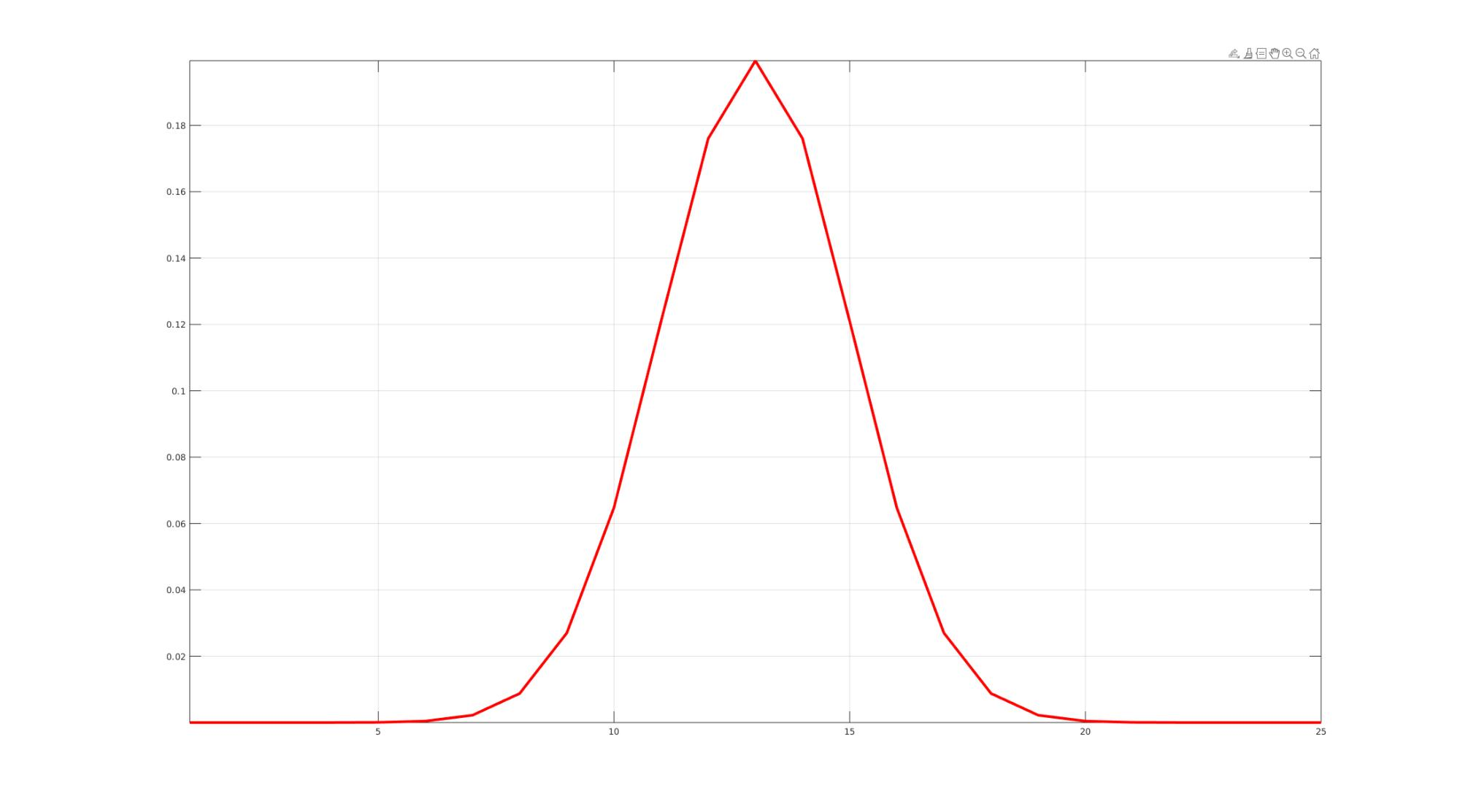## Example 2: 1D 'width' method

```k=25;
nd=1;
f=2;
methodOption='width';
hg=gauss_kernel(k,nd,f,methodOption);
```
```cFigure;
plot(hg,'r-','LineWidth',lineWidth);
axis tight; box on; grid on;
drawnow;
```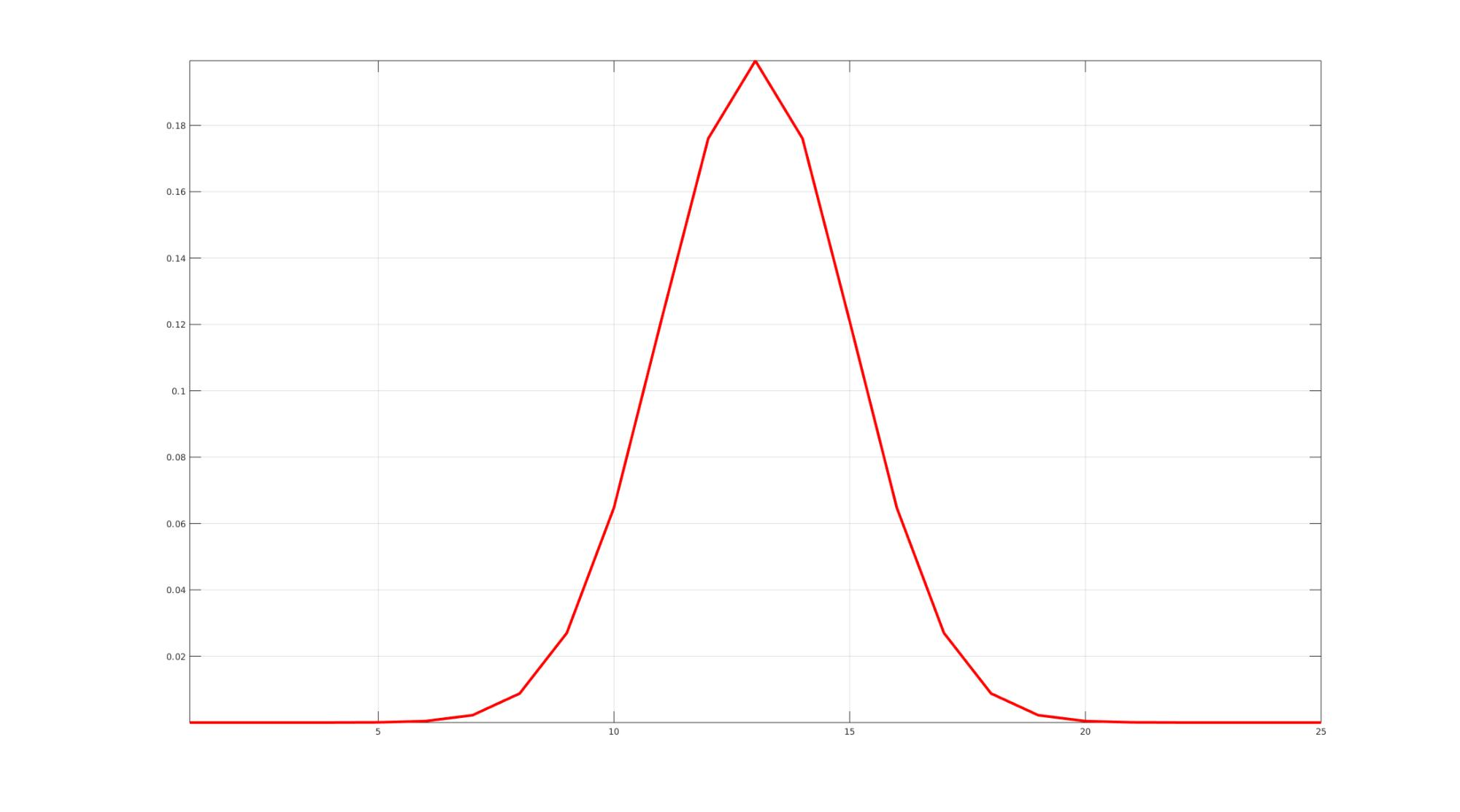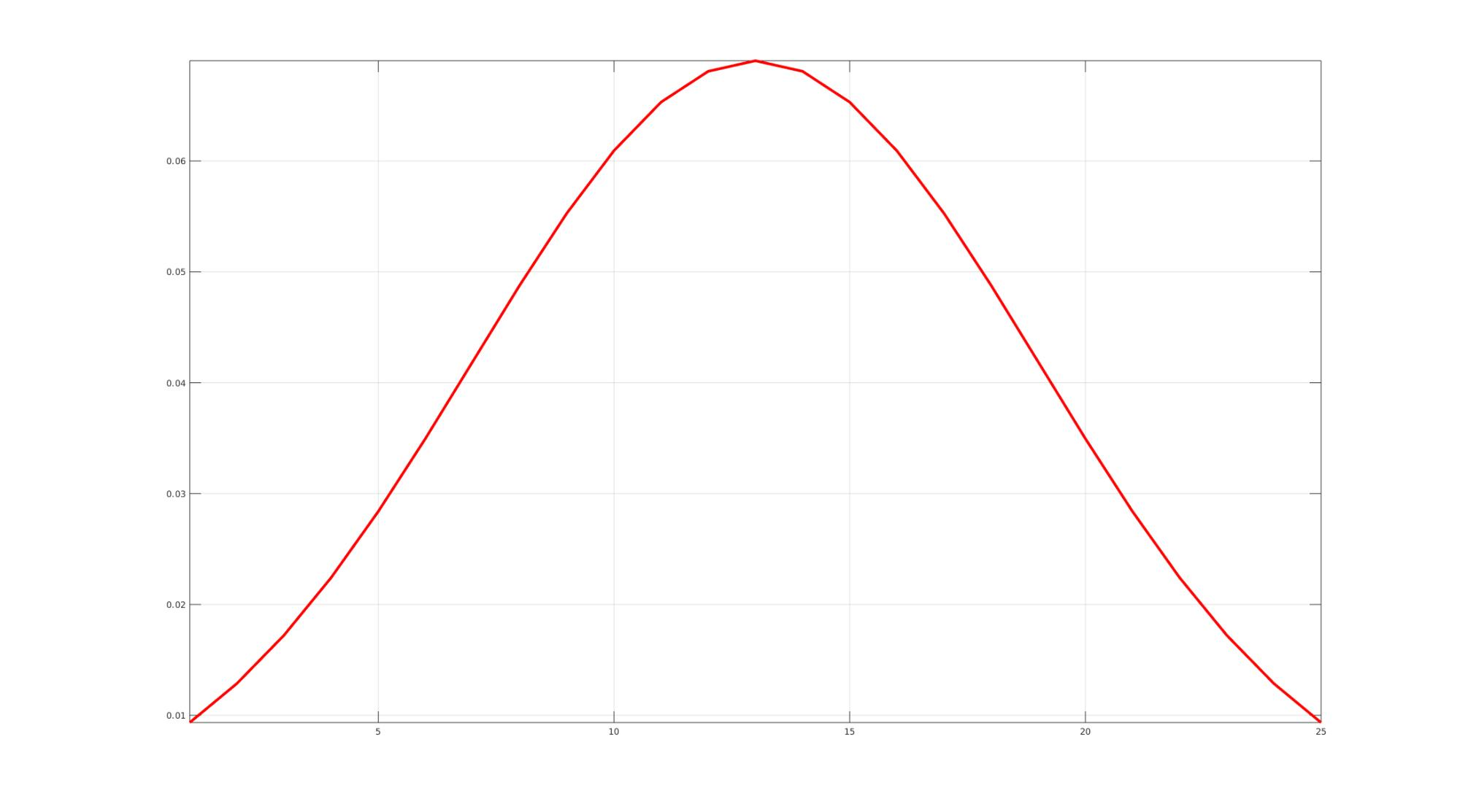## Example 3: 2D 'width' method

```k=11;
nd=2;
f=2;
methodOption='width';
hg=gauss_kernel(k,nd,f,methodOption);
```
```cFigure;
imagesc(hg);
axis tight; axis equal; box on;
colormap spectral; colorbar;
drawnow;
```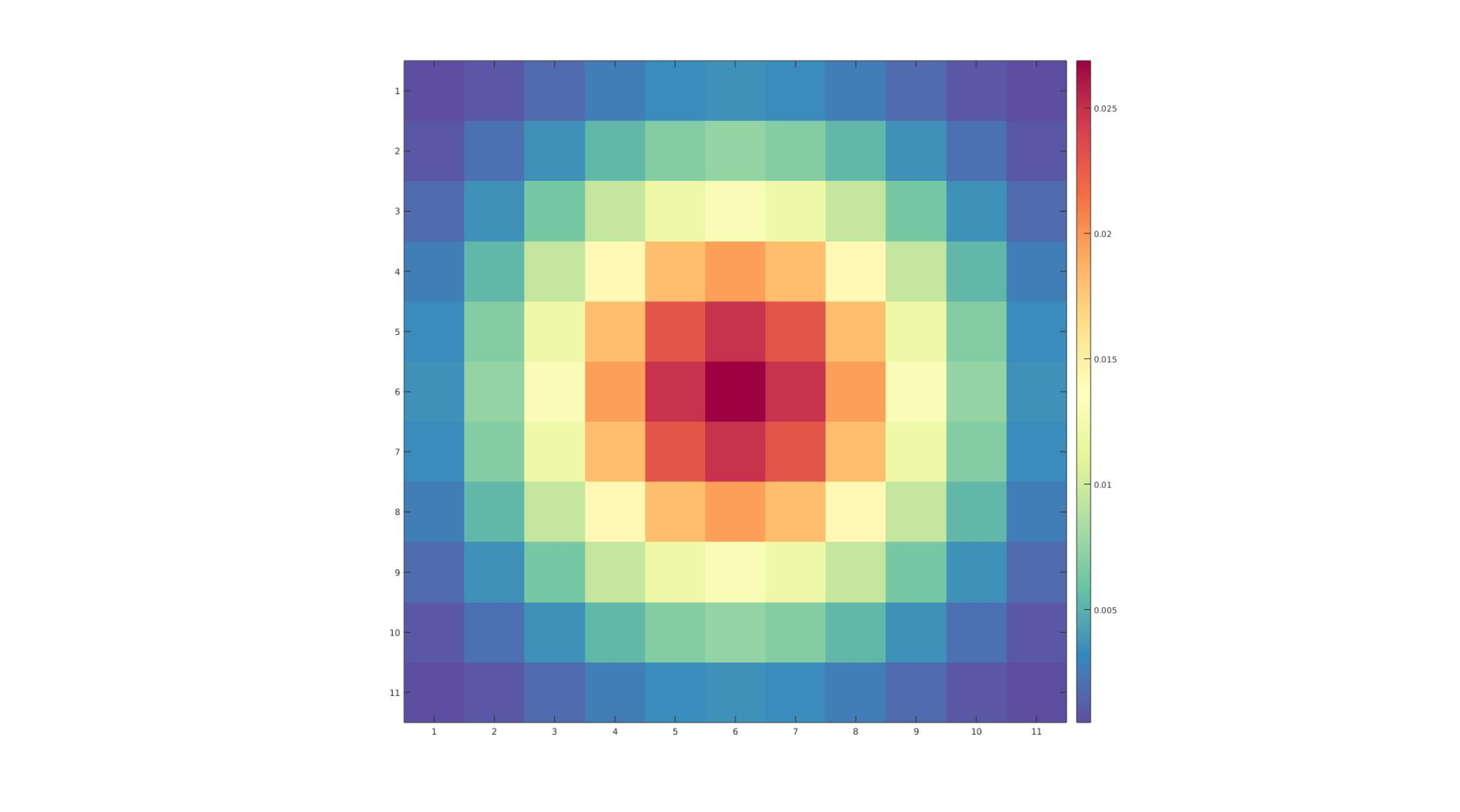## Example 4: 3D 'width' method

```k=11;
nd=3;
f=2;
methodOption='width';
hg=gauss_kernel(k,nd,f,methodOption);
```
```optionStruct.colormap=spectral(250);
sv3(hg,ones(1,3),optionStruct);
```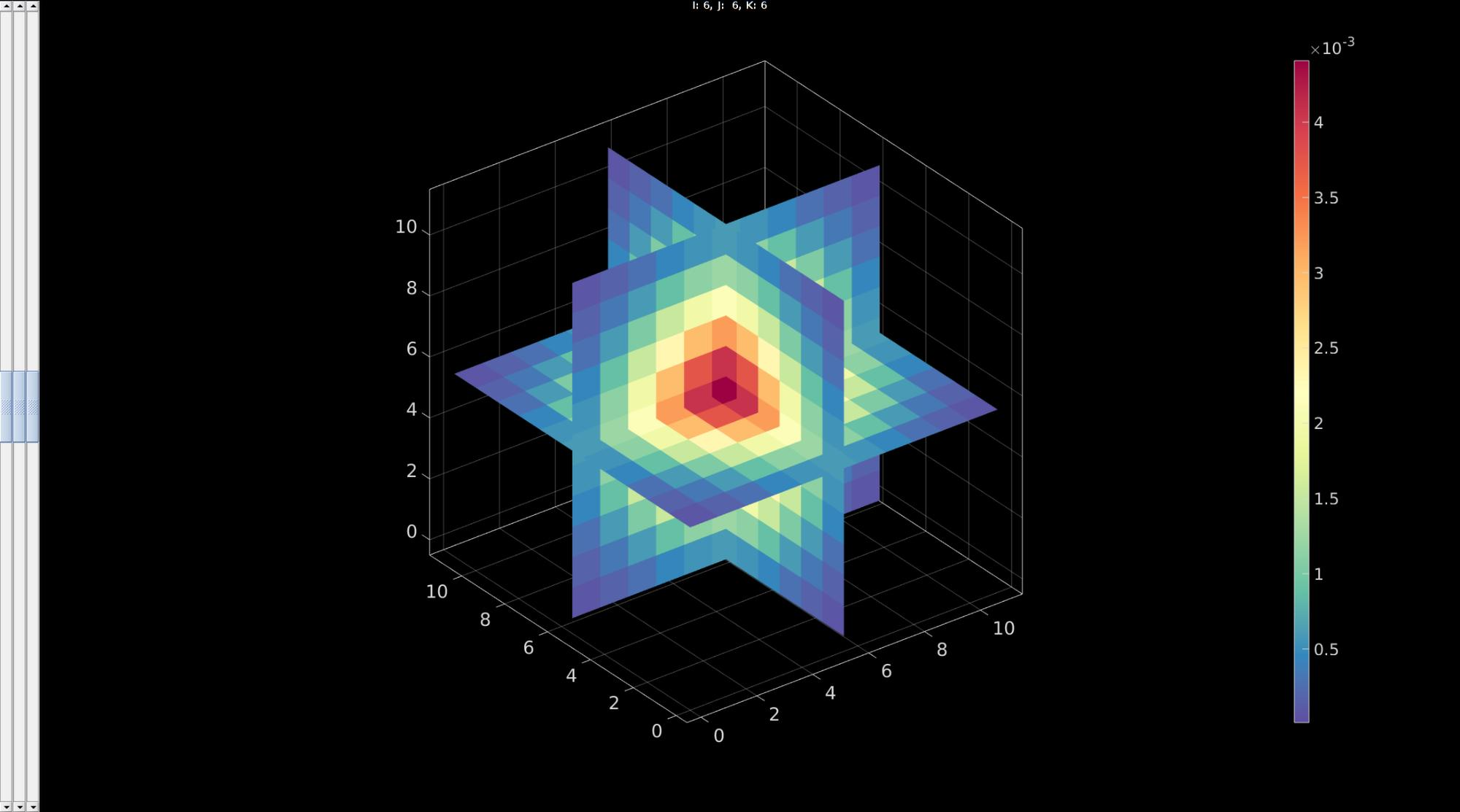GIBBON www.gibboncode.org

Kevin Mattheus Moerman, [email protected]

GIBBON footer text

GIBBON: The Geometry and Image-based Bioengineering add-On. A toolbox for image segmentation, image-based modeling, meshing, and finite element analysis.

Copyright (C) 2006-2023 Kevin Mattheus Moerman and the GIBBON contributors

This program is free software: you can redistribute it and/or modify it under the terms of the GNU General Public License as published by the Free Software Foundation, either version 3 of the License, or (at your option) any later version.

This program is distributed in the hope that it will be useful, but WITHOUT ANY WARRANTY; without even the implied warranty of MERCHANTABILITY or FITNESS FOR A PARTICULAR PURPOSE. See the GNU General Public License for more details.

You should have received a copy of the GNU General Public License along with this program. If not, see http://www.gnu.org/licenses/.